# SAT Math Multiple Choice Question 14: Answer and Explanation

### Test Information

Question: 14

4. rx2 =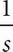x + 3

A quadratic equation is provided above, where r and s are constants. What are the solutions for x ?

• A.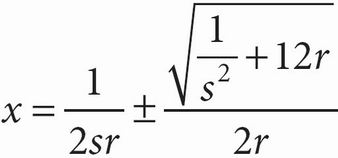• B.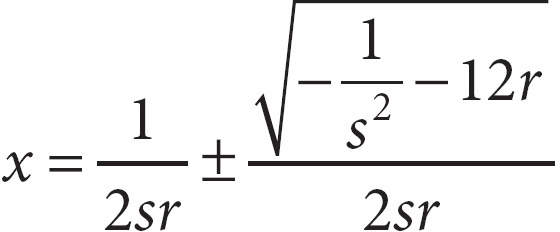• C.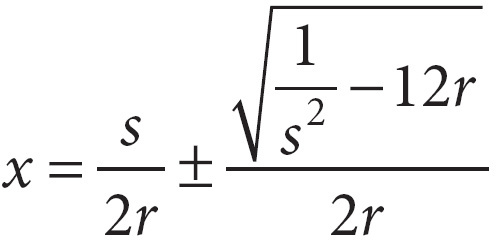• D.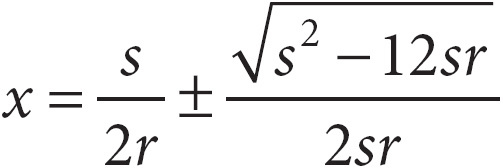A The first step to answering this question is to get the equation into the standard form of a quadratic equation by moving all the terms to the left or right side of the equation and setting it equal to zero, like this: rx3 -x - 3 = 0. Now that you have the equation in standard form, you can begin to solve for the roots. Since you are given variables instead of numbers, factoring this quadratic would require higher-level math, if it were even possible. You may have noticed the familiar form of the answer choices. They are in a form similar to the quadratic equation. Remember that a quadratic in standard form is represented by the equation ax2 + bx + c = 0, and the quadratic formula is x =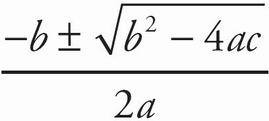. In this equation, a = r, b = -, and c = -3. Therefore,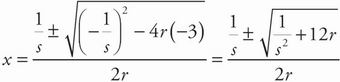. This exact format is not present in the answer choices, but the root part only matches the one in (A), so that is likely the answer. You will have to do a little more manipulation before you can get the equations to match exactly. The fractions need to be split up, so rewrite the equation as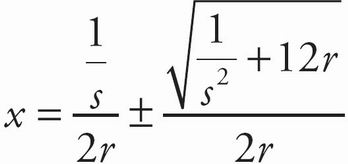or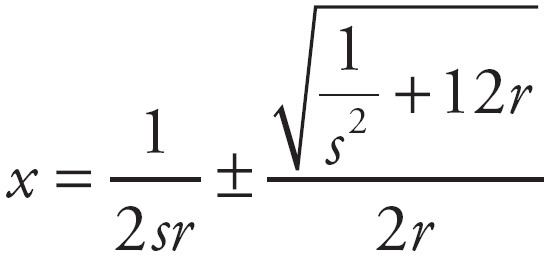.